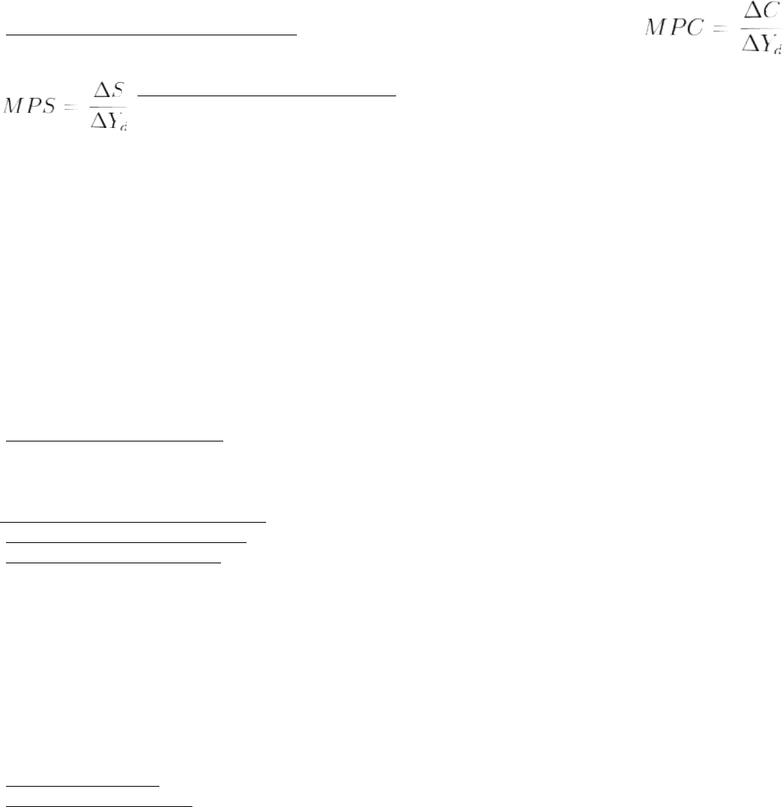Study Guides (380,000)
CA (150,000)
UW (6,000)
ECON (400)
ECON102 (100)
bean (8)

# ECON102 Study Guide - Aggregate Demand, Chapter 27, Consumption Function

Department
Economics
Course Code
ECON102
Professor
bean

This preview shows pages 1-2. to view the full 6 pages of the document.ECON 102: Chapter 27
Expenditure Multipliers: Keynesian Model
Fixed Prices and Expenditure Plans
-in the Keynesian Model all firms set their prices and sell the quantities their customers are willing to buy
-if the firms continuously sell more than they plan to and are running out of inventory, they will raise
their prices
-if the firms continuously sell more than they plan to and have excess inventory, they will lower their
prices
-on any given day the prices are fixed and the quantities the firms sell depend on demand not supply
-because of this, for the economy as a whole:
1. the price level is fixed
2. aggregate demand determines real GDP
-the Keynesian Model explains fluctuations in aggregate demand at a fixed price level by identifying the forces
that determine expenditure plans
Expenditure Plans
-aggregate expenditure has four components: consumption expenditure, investment, government expenditure
on goods and services and net exports
-these four components sum to real GDP
-aggregate planned expenditure: equal to the sum of the planned levels of consumption expenditure,
investment, government expenditure on goods and services and exports minus imports
-two of these components (consumption expenditure and imports) change when income changes and
so they depend on real GDP
A Two-Way Link Between Aggregate Expenditure and Real GDP
-all else remaining the same:
-an increase in real GDP increased aggregate expenditure
-an increase in aggregate expenditure increases real GDP
Consumption and Saving Plans
-the most important factors that influence consumption expenditure are:
1. disposable income
2. real interest rate
3. wealth
4. expected future income
-disposable income: aggregate income minus taxes plus transfer payments
-aggregate income equals real GDP so disposable income depends on real GDP
Consumption Expenditure and Saving
-households can only spend their disposable income on consumptions or save it, so planned
consumption expenditure plus planned saving always equals disposable income
-consumption function: the relationship between consumption expenditure and disposable income
-saving function: the relationship between saving and disposable income
Consumption Function
-disposable income is on the x-axis and consumption expenditure is on the y-axis
-as disposable income increases, so does consumption expenditure
-autonomous consumption: the amount of consumption that would take place in the short run even if
people had no current income (when disposable income is zero; y-intercept)
-induced consumption: consumption expenditure in excess of the autonomous amount; consumptions
expenditure that is induced by an increase in disposable income
45o Line
-the line showing disposable income
-when this line is above consumption expenditure, disposable income is greater than consumption
expenditure
-the reverse is true when this line is below consumption expenditure
Saving Function

Only pages 1-2 are available for preview. Some parts have been intentionally blurred.-as disposable income increases, savings increases
-when consumption expenditure exceeds disposable income saving is negative
Marginal Propensities to Consume and Save
-marginal propensity to consume (MPC): the fraction of a change in disposable
income that is spend on consumption
-calculated as the change in consumption expenditure divided by the change in disposable income
- marginal propensity to save (MPS): the fraction of a change in disposable income that is
saved
-calculated as the change in saving divided by the change in disposable income
-because an increase in disposable income is either spent or saved, the marginal propensity to consume plus
the marginal propensity to save equals 1
-MPS + MPC = 1
Slopes and Marginal Propensities
-the slope of the consumption function is the marginal propensity to consume
-the slope of the saving function is the marginal propensity to save
Consumption as a Function of Real GDP
-consumption expenditure changes when disposable income changes and disposable income changes when
real GDP changes
-consumption expenditure depends not only on disposable income but also on real GDP
Import Function
-an increase in Canadian real GDP increases the quantity of Canadian imports
-marginal propensity to import: the relationship between imports and real GDP
-the fraction of an increase in real GDP that is spend on income
-calculated as the change in imports divided by the change in real GDP
Real GDP with a Fixed Price Level
-aggregate expenditure schedule: lists aggregate planned expenditure generated at each level of real GDP
-aggregate expenditure curve: graph of the aggregate expenditure schedule
Aggregate Planned Expenditure
-see page 653 for table and graph example
-to calculate aggregate planned expenditure at a given real GDP we add the expenditure components together
-investment and government expenditure on goods and services are independent of the level of real GDP
-investment depends on the real interest rate and the expected profit
-exports are influenced by events in the rest of the world, prices of foreign-produced goods and services
relative to the prices of similar Canadian produced goods and services, and exchange rates
-exports are not directly affected by Canadian real GDP
-aggregate expenditure curve has real GDP on the x-axis and aggregate planned expenditure on the y-axis
-to construct this curve (also known as the AE curve) subtract imports from I + G + X + C
-induced expenditure: consumption expenditure minus imports, which varies with real GDP
-autonomous expenditure: the sum of investment, government expenditure, and exports which doesn’t vary
with real GDP
-another way of think about this expenditure is that it is the level of aggregate planned expenditure if
real GDP were zero
-aggregate expenditure curve summarizes the relationship between aggregate planned expenditure and real
GDP
Actual Expenditure, Planned Expenditure and Real GDP
-actual aggregate expenditure is always equal to real GDP
-aggregate planned expenditure isn’t always equal to actual aggregate expenditure and therefore isn’t always
equal to real GDP
-the reason for this is due to the fact that firms can end up with inventories that are greater or smaller
than planned